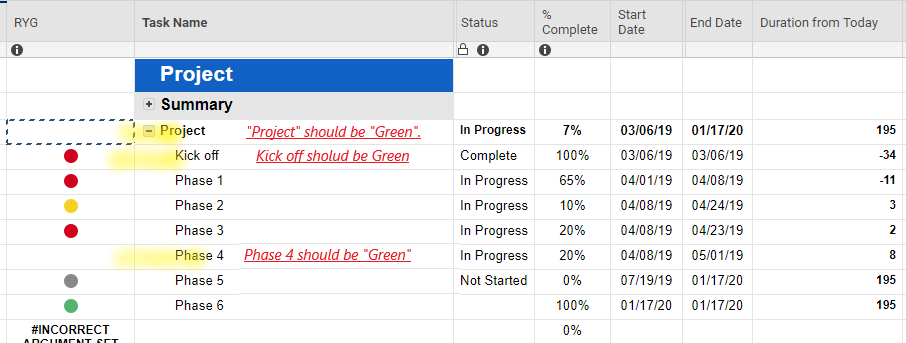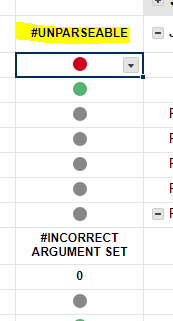or Explore Discussions

# Formula RYG automation with using Complete% and rest of date by end date.

04/19/19 Edited 12/09/19

hi

I'm trying to create Formula. It's almost completed. However, one last  rule doesn't work.

Can someone suggest to modify my formula?

Currently the following conditions and formula is working fine.

-  If Complete% is 100% = Green

- If Complete% is upper 60%  = Green

- if Complete% is under 60% and Today is less 5 days before End date = Yellow

- if Complete% is under 60% and Today is less 3 days before End date OR behind End date = Red

- If Complete% is 0 = Gray

=IF(OR([% Complete]1 = 1, [% Complete]1 > 0.6), "Green", IF(AND([% Complete]1 < 0.6, [% Complete]1 > 0), IF(NETWORKDAYS(TODAY(), [End Date]13) < 5, IF(NETWORKDAYS(TODAY(), [End Date]1) <= 3, "Red", "Yellow"), "Green"), "Gray"))

but I tried to add one more rule, then it doesn't work, error said "UNPARSEABLE"

wanted to add one more rule is

- Even if complete% is upper 60%, Today is less 2 days before End date = Red

=IF(OR([% Complete]1 = 1, (AND([% Complete]1 > 0.6, (NETWORKDAYS(TODAY(), [End Date]1) > 3)))), "Green", IF(AND([% Complete]1 < 0.6, [% Complete]1 > 0), IF(NETWORKDAYS(TODAY(), [End Date]1) < 5, IF(NETWORKDAYS(TODAY(), [End Date]1) <= 3, "Red", "Yellow"), "Green"), "Gray")))

• Let's see if we can't simplify this a little bit and work in your extra criteria...

Working Formula:

=IF(OR([% Complete]1 = 1, [% Complete]1 > 0.6), "Green", IF(AND([% Complete]1 < 0.6, [% Complete]1 > 0), IF(NETWORKDAYS(TODAY(), [End Date]13) < 5, IF(NETWORKDAYS(TODAY(), [End Date]1) <= 3, "Red", "Yellow"), "Green"), "Gray"))

Simplified Formula:

=IF([% Complete]1 > 0.6, "Green", IF([% Complete]1 > 0, IF(NETWORKDAYS(TODAY(), [End Date]1) < 3, "Red", IF(NETWORKDAYS(TODAY(), [End Date]1) < 5, "Yellow")), IF([% Complete]1 = 0, "Gray")))

Simplified Formula + New Criteria:

=IF([% Complete]1 > 0.6, IF(NETWORKDAYS(TODAY(), [End Date]1) < 2, "Red", "Green"), IF([% Complete]1 > 0, IF(NETWORKDAYS(TODAY(), [End Date]1) < 3, "Red", IF(NETWORKDAYS(TODAY(), [End Date]1) < 5, "Yellow")), IF([% Complete]1 = 0, "Gray")))

thinkspi.com

• hi, Paul. Thank you so much for your kindly suggestion and created new formula. Appreciate that. Then, I checked it if it's working properly or not. Most rule does work.

but the below criteria for "Green" doesn't work well.

- Complete% gets 100% = "Green"

- Complete% is under 60% but today is over 5 days remaining before End date = "Green"

2nd criteria might be difficult to be added in current formula. But i wanted to add 1st one at least otherwise, all 100% completed task is going to be Red.

I tried to add criteria by using "OR". but my formula is wrong to work.

=IF((OR[% Complete]1 = 1, [% Complete]1 > 0.6), IF(NETWORKDAYS(TODAY(), [End Date]1) < 2, "Red", "Green"), IF([% Complete]1 > 0, IF(NETWORKDAYS(TODAY(), [End Date]1) < 3, "Red", IF(NETWORKDAYS(TODAY(), [End Date]1) < 5, "Yellow")), IF([% Complete]1 = 0, "Gray")))• =IF(([% Complete]1 = 1, "Green", IF([% Complete]1 > 0.6, IF(NETWORKDAYS(TODAY(), [End Date]1) < 2, "Red", "Green"), IF([% Complete]1 > 0, IF(NETWORKDAYS(TODAY(), [End Date]1) < 3, "Red", IF(NETWORKDAYS(TODAY(), [End Date]1) < 5, "Yellow"), "Green"), IF([% Complete]1 = 0, "Gray"))))

My apologies. Give this a try...

thinkspi.com

• hi, Thanks for your support. Appreciate that.

But unfortunately that doesn't work still. "Unparseable" .

Yellow line was error that i pasted your new formula. Do you think if you still modify any by new idea? Thank you again for your help• That's my fault. I just copy/pasted your formula and made adjustments to the criteria. I completely missed a few extra parenthesis that had been tucked in.

=IF([% Complete]1 = 1, "Green", IF([% Complete]1 > 0.6, IF(NETWORKDAYS(TODAY(), [End Date]1) < 2, "Red", "Green"), IF([% Complete]1 > 0, IF(NETWORKDAYS(TODAY(), [End Date]1) < 3, "Red", IF(NETWORKDAYS(TODAY(), [End Date]1) < 5, "Yellow"), "Green"), IF([% Complete]1 = 0, "Gray"))))

thinkspi.com